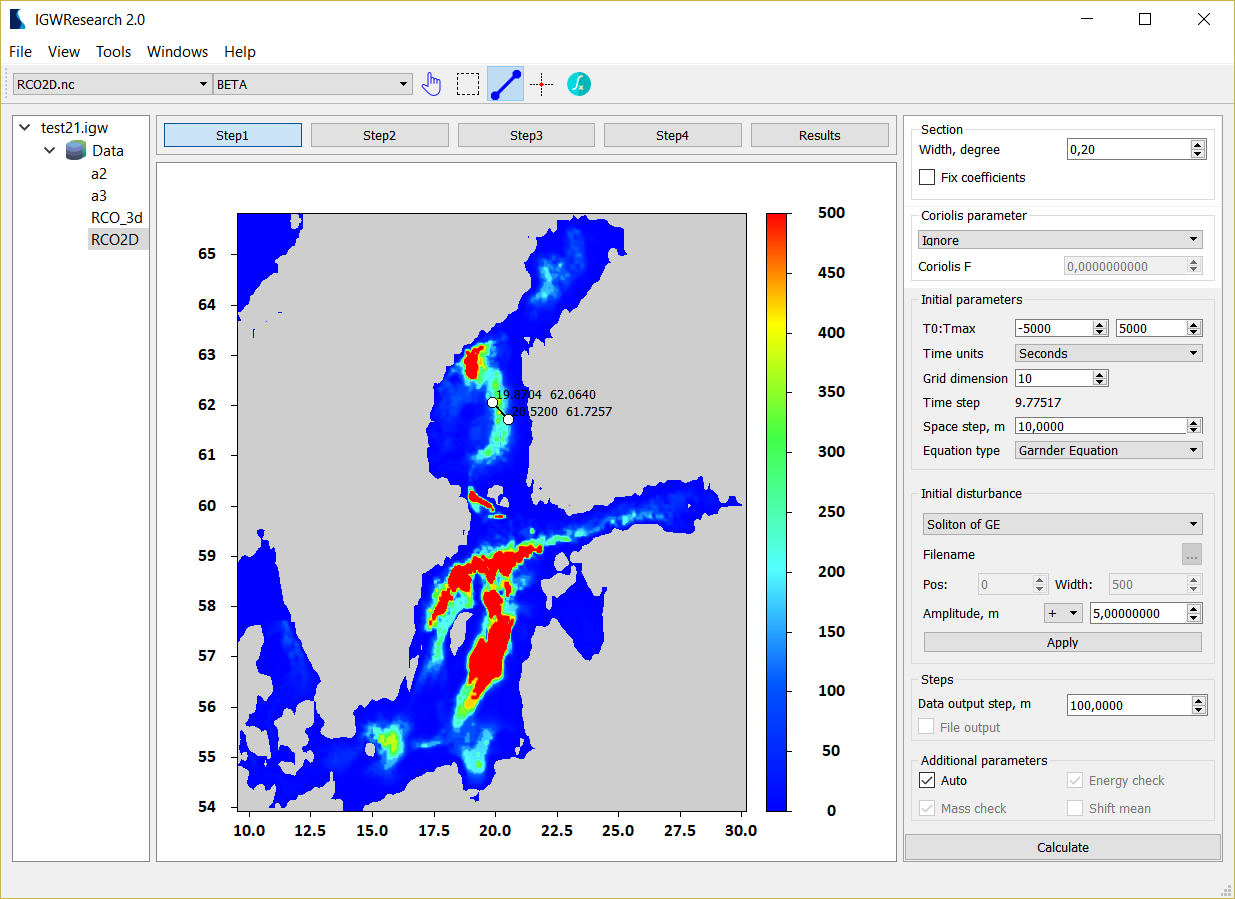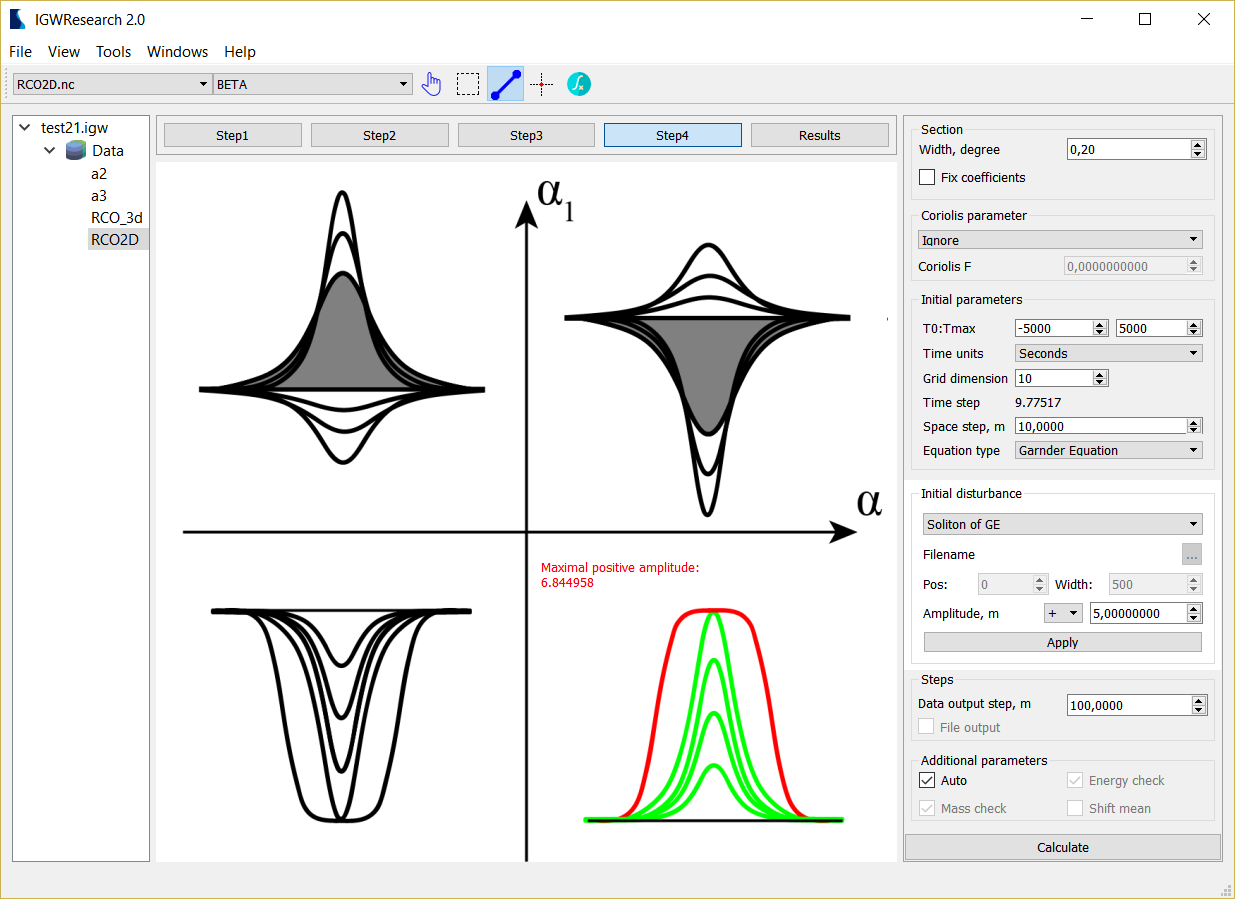The application of mathematical modeling for the study of geophysical processes is one of the main tools in the study of large-scale natural phenomena. One of these phenomena is the propagation and transformation of internal waves in the ocean. Such waves arise in the water column under the influence of gravity and due to the stratification of water by salinity and temperature with a change of depth. Internal waves are a topical object of research as they make a significant contribution to the formation of the bottom relief and the impact on the supports of hydraulic structures, which directly affects both the economic activities of man on the shelf and the ecosystem as a whole.
During the implementation of the first stage, the following steps were performed: designing a software package, developing calculation blocks and algorithms for processing multidimensional data (representing physical fields distributed on a geographic grid), algorithms for loading data into the software, algorithms for constructing and preparing data samples for mathematical modeling, a number of numerical models (the Korteweg-de Vries equation, the Gardner equation, the Gardner-Ostrovsky equation) for modeling transformation of the internal waves, a model for particles trajectory calculation, and a number of software tools for visualizing and analyzing results.
To initialize numerical models in the software package, the density data obtained from open data sources using the equation of state of sea water based on the hydrological atlases WOA13, GDEM is used. To specify the shoreline, a bathymetry atlas ETOPO1 is used.

The project is supported by the Russian Science Foundation (project № 17-71-10101)